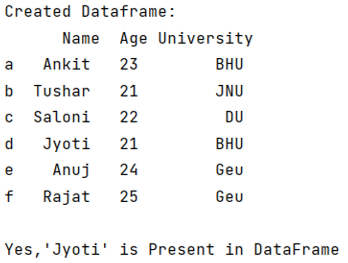# How to determine whether a Pandas Column contains a particular value?

Given a Pandas DataFrame, we have to determine whether its Column contains a particular value. By Pranit Sharma Last updated : September 20, 2023

Pandas is a special tool which allows us to perform complex manipulations of data effectively and efficiently. Inside pandas, we mostly deal with a dataset in the form of DataFrame. DataFrames are 2-dimensional data structure in pandas. DataFrames consists of rows, columns and the data.

Columns are the different fields that contain their particular values when we create a DataFrame. We can perform certain operations on both rows & column values. Here, we are going to check the whether a value is present in a column or not.

## Problem statement

Given a Pandas DataFrame, we have to determine whether its Column contains a particular value.

## Determining whether a Pandas Column contains a particular value?

For this purpose, we will use a simple python keywords 'in' & 'not in'. These keywords are used to check whether a value is present in a series or collection or not.

Let us understand with the help of an example,

## Python program to determine whether a Pandas Column contains a particular value

```# Import pandas Package
import pandas as pd

# Creating dictionary
d = {
'Name':['Ankit', 'Tushar', 'Saloni','Jyoti', 'Anuj', 'Rajat'],
'Age':[23, 21, 22, 21, 24, 25],
'University':['BHU', 'JNU', 'DU', 'BHU', 'Geu', 'Geu']
}

# Creating a Dataframe
df = pd.DataFrame(d,index = ['a', 'b', 'c', 'd', 'e', 'f'])

print("Created Dataframe:\n", df)
# check 'Jyoti' exist in DataFrame or not

if 'Jyoti' in df.values :
print("\nYes,'Jyoti' is Present in DataFrame")
else :
print("\nSorry!, This value does not exists in Dataframe")
```

### Output

The output of the above program is: